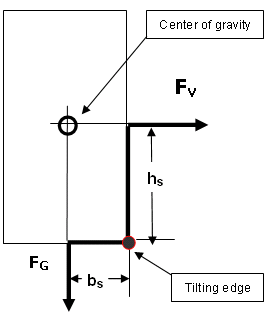1.4.2  Tipping loads / risk of tipping [German version]

Horizontal acceleration may also cause packages to tip.

A package is at risk of tipping if the area of contact with the floor is small and the center of gravity is high after due consideration of the acceleration to be expected. This is the case, for instance, if the center of gravity is above half the height of the package.

To determine whether packages are at risk of tipping, the ratio between the height of the center of gravity and the distance to the tilting edge must be determined. The tilting edge is always the edge perpendicular to the direction of acceleration (see Figure 2).FG = weight force FV = acceleration force hs = tipping moment arm bs = resting moment arm

Figure 2: Checking the risk of tipping

Whenever the ratio of the height of the center of gravity over the base to the lateral distance between the tilting edge and the center of gravity is larger than the expected acceleration forces, the box is at risk of tipping and must be secured accordingly. Expressed in terms of ratios, this means:to the front if bs / hs < 1.0*to the side if bs / hs < 0.5*to the rear if bs / hs < 0.5*

* Example acceleration values during transportation by truck
(CTU Packing Guidelines, see Table 1 in section 1.3.1).